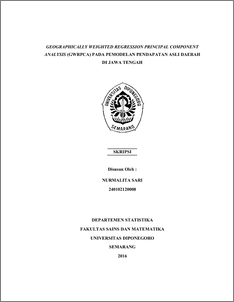# GEOGRAPHICALLY WEIGHTED REGRESSION PRINCIPAL COMPONENT ANALYSIS (GWRPCA) PADA PEMODELAN PENDAPATAN ASLI DAERAH DI JAWA TENGAH

SARI, NURMALITA (2016) GEOGRAPHICALLY WEIGHTED REGRESSION PRINCIPAL COMPONENT ANALYSIS (GWRPCA) PADA PEMODELAN PENDAPATAN ASLI DAERAH DI JAWA TENGAH. Undergraduate thesis, Fakultas Sains dan Matematika, Undip.Preview
PDF
3074Kb

## Abstract

Linear Regression Analysis is a method for modeling the relation between a response variable with two or more independent variables. Geographically Weighted Regression (GWR) is a development of the regression model where each observation location has different regression parameter values because of the effects of spatial heterogenity. Regression Principal Component Analysis (PCA) is a combination of PCA and are used to remove the effect of multicolinearity in regression. Geographically Weighted Regression Principal Component Analysis (GWRPCA) is a combination of PCA and GWR if spatial heterogenity and local multicolinearity occured. Estimation parameters for the GWR and GWRPCA using Weighted Least Square (WLS). Weighting use fixed gaussian kernel function through selection of the optimum bandwidth is 0,08321242 with minimum Cross Validation (CV) is 3,009035. There are some variables in PCA that affect locally-generated revenue in Central Java on 2012 and 2013, which can be represented by PC1 that explained the total variance data about 71,4%. GWRPCA is a better model for modeling locally-generated revenue for the districts and cities in Central Java than RPCA because it has the the smallest Akaike Information Criterion (AIC) and the largest R2.. Keywords : Spatial Heterogenity, Local Multicolinearity, Principal Component Analysis, Geographically Weighted Regression Principal Component Analysis.

Item Type: Thesis (Undergraduate) H Social Sciences > HA Statistics Faculty of Science and Mathematics > Department of Statistics 55040 Mr Hasbi Yasin 25 Jul 2017 14:08 25 Jul 2017 14:08

Repository Staff Only: item control page